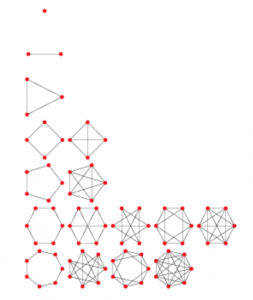深度学习折腾记（1）－Python环境配置

https://it.stonybrook.edu/help/kb/installing-software-packages-locally-with-anaconda

正规图(Regular Graph)数量的统计

In graph theory, a regular graph is a graph where each vertex has the same number of neighbors; i.e. every vertex has the same degree or valency. A regular directed graph must also satisfy the stronger condition that the indegree and outdegree of each vertex are equal to each other. A regular graph with vertices of degree k is called a k‑regular graph or regular graph of degree k. Also, from the handshaking lemma, a regular graph of odd degree will contain an even number of vertices.Regular图的示意（来源：http://mathworld.wolfram.com/RegularGraph.html）

http://oeis.org/wiki/User:Jason_Kimberley/A068934

40K36

genreg95下载地址